# Plane sailing

When we refer to plane sailing we mean sailing from one position to another where the latitude and longitude of each is different to the other.  Because we are using a mean latitude, this method is not very accurate over long distances and should be confined to distances of 600 nautical miles or less.  To determine course and distance with this method we make use of three formulas: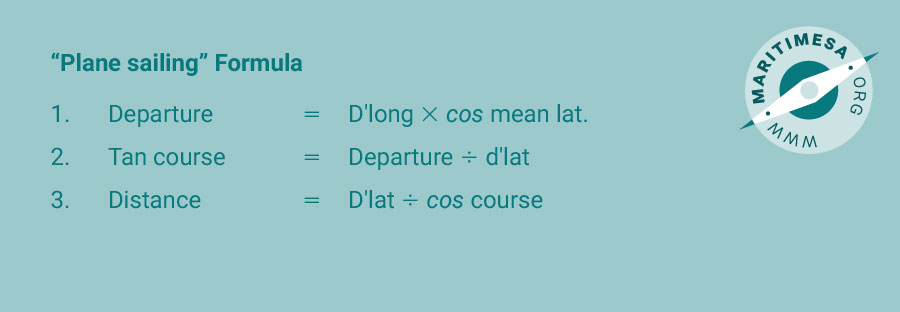The double triangle in the following sketch shows how these formulae were derived: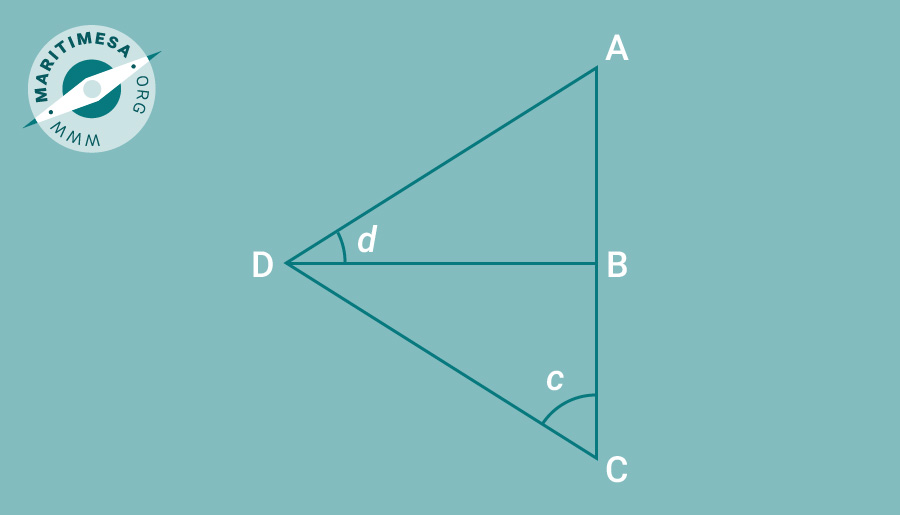Side AD is the d’long, side DB is the departure, angle ADB is the m’lat. Side DC is the distance, side BC is the d’lat and angle BCD is the azimuth.

In order to solve for the course and distance we must first determine the departure by using formula (1).
Since there are two latitudes we need to find an approximation, ie the numerical mean between the two. For example: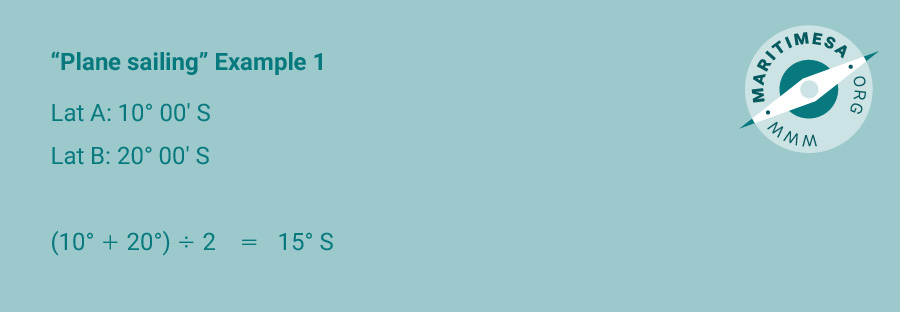Since they are named the same (both south), we simply add the two together and divide by 2 and name the mean lat the same as the two, ie south.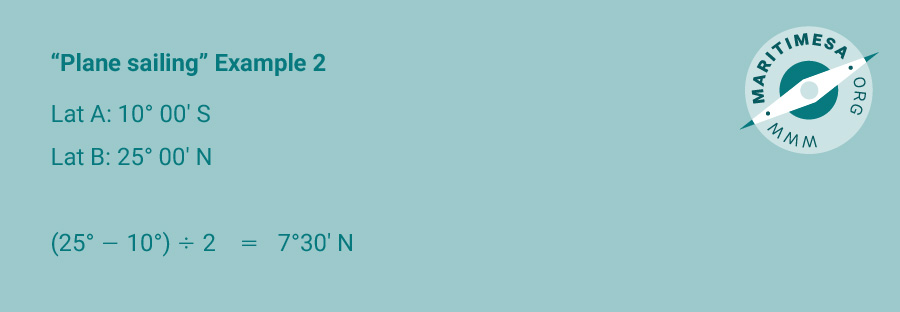Since they are named differently, we cannot use the above method. We therefore need to subtract the smaller from the larger, divide the result by 2 and name the quantity so derived the same as the larger of the two.

Once we have the departure we can then use the 2nd formula to determine the course to be steered, i.e.
Tan course = Departure / d’lat

The answer you get from your calculator will not be the true course, but the azimuth. Remember that an azimuth is always measured from the north/south line (meridian),ie it will lie in one of the four quadrants: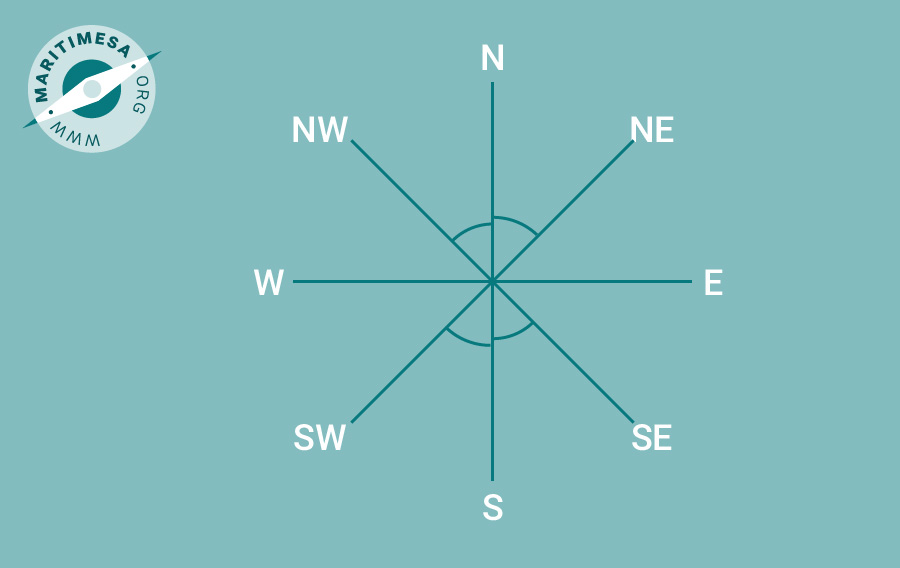To convert to a true course you should do one of the following:
N xxx° E – xxx° east of north – 000° + xxx°
N xxx° W – xxx° west of north – 360° – xxx°
S xxx° W – xxx° west of south – 180° + xxx°
S xxx° E – xxx° east of south – 180° – xxx°

Once you have the azimuth and true course you can work out the distance to be steamed by using the 3rd formula, i.e.
Distance = D.lat / cos course
Although the formula says ‘cos course’ it should read ‘cos azimuth’.
As a guide a pro-forma of the method used to determine course and distance using the Plane sailing method is shown below as appendix C, titled “PLANE SAILING”.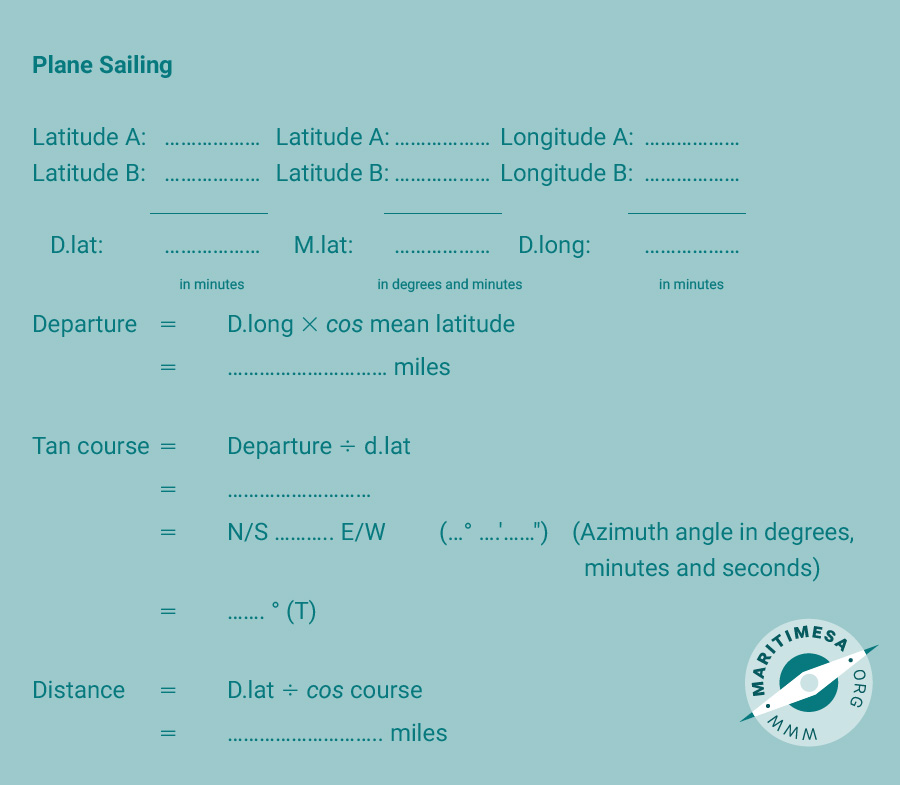Appendix C